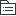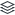# 五脏

|
7

## 相关模板推荐

•## 五脏 —— 作品大纲

•• 心的解剖形态
• 心的生理功能
• 心主血脉
• 血：血液；脉：经脉
• 行血以运送营养物质；生血使血液得到补充
• 心主神志
• 心藏神，肺藏魄，肝藏魂，脾藏意，肾藏志
• 心的生理特性
• 心为阳脏而主阳气
• 心气与夏气相应
• 心包络
• 保护心脏，代心受邪的作用
•• 肺的解剖形态
• 肺的生理功能
• 肺主气
• 肺主呼吸之气
• 肺主一身之气
• 肺主行水
• 肺气宣发
• 散布全身
• 肺气肃降
• 生成尿液
• 肺主治节
• 肺主呼吸
• 调节气机
• 助心行血
• 宣发肃降
• 肺主宣肃
• 宣发
• 吸清呼浊
• 输布津液精微
• 宣发卫气
• 肃降
• 吸入清气
• 输布津液精微
• 通调水道
• 清肃结晶
• 肺的生理特性
• 肺为华盖
• 肺为娇脏
• 肺气与秋气相应
•• 脾的解剖形态
• 脾的生理功能
• 脾主运化
• 运化水谷
• 消化水谷
• 吸收转输精微
• 将精微转化为气血
• 运化水湿
• 脾主生血统血
• 脾主升清
• 胃之降浊
• 脾的生理特性
• 宜升则健
• 喜燥恶湿
• 脾气与长夏相应
•• 肝的解剖形态
• 肝的生理功能
• 肝主疏泄
• 调畅气机
• 调节精神情志
• 促进消化吸收
• 维持气血运行
• 调节水液代谢
• 调节性与生殖
• 肝藏血生血
• 藏血
• 贮藏血液
• 调节血量
• 生血
• 肝的生理特性
• 喜调达
• 体阴而用阳
• 肝为刚脏
• 肝气与春气应
•• 肾的解剖形态
• 肾的生理功能
• 肾藏精
• 精的含义
• 先天之精
• 后天之精
• 精的生理功能
• 促进生殖繁衍
• 促进生长发育
• 参与血液生成
• 抵御外邪侵袭
• 肾主水液
• 将水谷精微中具有濡养滋润脏腑组织作用的津液输布周身
• 将各脏腑组织代谢利用后的浊液排出体外
• 肾主纳气
• 肾主一身阴阳
• 肾精
• 肾气
• 肾阴
• 肾阳
• 肾的生理特性
• 肾主封藏
• 藏精
• 纳气
• 主水
• 固胎
• 肾气与秋气应
• 命门
• 命门的位置
• 命门的功能
• 原气所系
• 藏精舍神
• 水火之宅
• 内愈真火